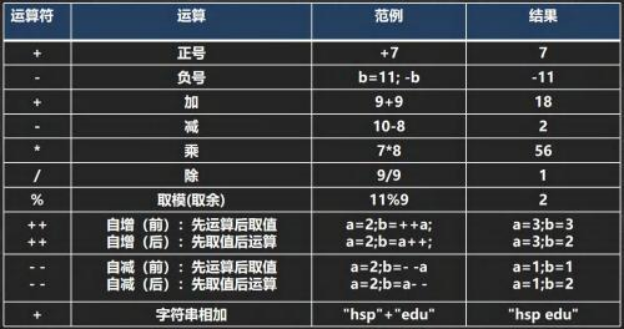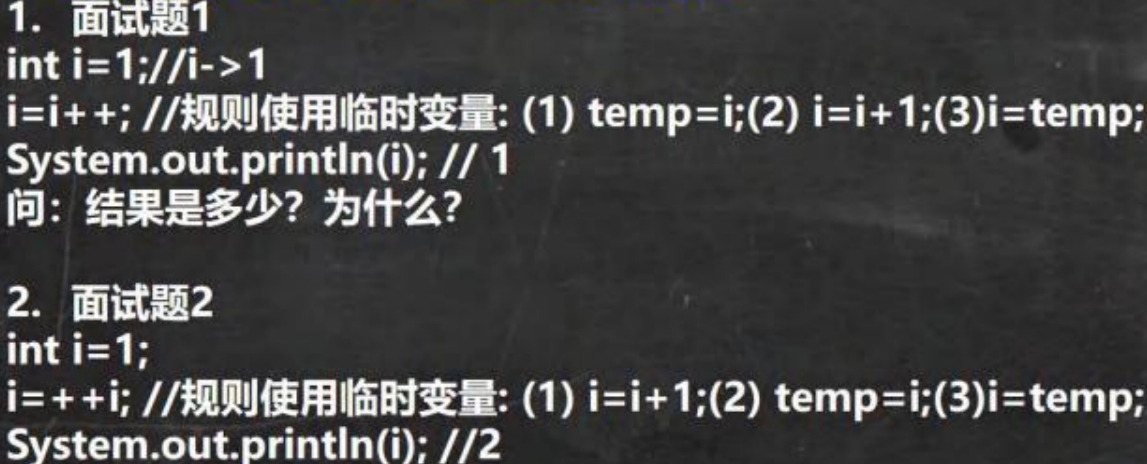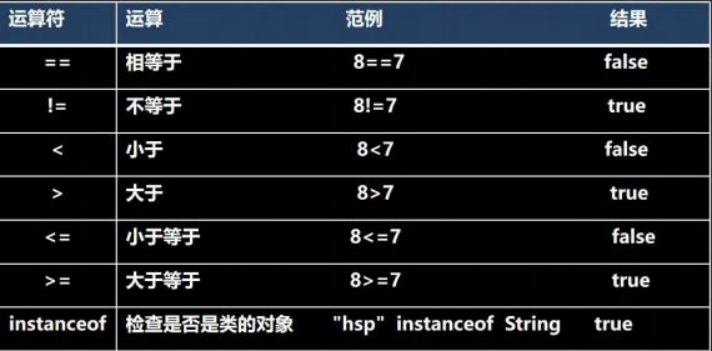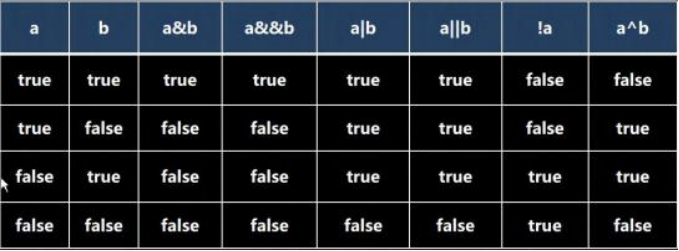# 运算符详解## 2、算术运算符介绍## 3、取模%的本质

% 是用来取余数的， 只能用在整数 取模 a%b的本质是： a % b = a - a / b * b

``````10 % 3 => - 10 - (- 10) / 3 * 3 = - 10 + 9 = - 1
10 % -3 = 10 - 10 / (-3) * (-3) = 10 - 9 = 1
10 % -3 =    (- 10) - (- 10) / (-3) * (-3) = - 10 + 9 = - 1``````

## 4.自增++

int j = 8; int k = ++j; //等价 j=j+1;k=j; int k = j++; // 等价 k =j;j=j+1;## 1、定义

1. 关系运算符的结果都是 boolean 型，也就是要么是true ，要么是 false
2. 关系表达式 经常用在 if 结构的条件中或循环结构的条件中

## 2、关系运算符介绍## 3、细节说明

1. 关系运算符的结果都是 boolean 型，也就是要么是 true ，要么是 false。
2. 关系运算符组成的表达式，我们称为关系表达式。 a > b
3. 比较运算符"==“不能误写成”="

## 2、逻辑运算符介绍

1. 短路与 && ， 短路或 || ，取反 !
2. 逻辑与 & ，逻辑或 | ，^ 逻辑异或## 3、运算规则

``````1)   a&b : &  叫逻辑与： 规则： 当 a  和 b  同时为 true ,则结果为 true,  否则为 false
2)   a&&b : &&  叫短路与： 规则： 当 a  和 b  同时为 true ,则结果为 true,否则为 false
3)   a|b : |  叫逻辑或，规则： 当 a  和 b  ，有一个为 true ,则结果为 true,否则为 false

4)   a||b : ||  叫短路或，规则： 当 a  和 b  ，有一个为 true ,则结果为 true,否则为 false
5)    !a :  叫取反，或者非运算。当 a  为 true,  则结果为 false,  当 a  为 false 是，结果为 true
6)   a^b:  叫逻辑异或，当 a  和 b  不同时，则结果为 true,  否则为 false``````

## 3、赋值运算符特点

1. 运算顺序从右往左 int num = a + b + c;
2. 赋值运算符的左边 只能是变量,右边 可以是变量、表达式、常量值 int num = 20; int num2= 78 * 34 - 10; int num3 = a;
3. 复合赋值运算符等价于下面的效果 比如： a+=3;等价于 a=a+3; 其他类推
4. 复合赋值运算符会进行类型转换。 byte b = 2; b+=3; b++;
``````byte b = 3;
b += 2;    等价 b = (byte)(b + 2);
b++;   b = (byte)(b+1);``````

## 1.基本语法

1. 如果条件表达式为true ，运算后的结果是表达式 1；
2. 如果条件表达式为false ，运算后的结果是表达式 2；

## 2.使用细节

1. 表达式 1 和表达式 2 要为可以赋给接收变量的类型(或可以自动转换)
2. 三元运算符可以转成 if–else 语句
``````int res = a > b ?    a++ :    --b;
if ( a > b) res = a++;
else res = --b;``````
``````//三元运算符细节

public class TernaryOperatorDetail {

//编写一个 main 方法
public static void main(String[] args) {
//表达式 1 和表达式 2 要为可以赋给接收变量的类型
//(或可以自动转换/或者强制转换)
int a = 3;
int b = 8;
int c = a > b ? (int)1. 1 : (int)3.4;//可以的
double d = a > b ? a : b + 3;//可以的，满足 int -> double
}
}``````

-- 展开阅读全文 --

« 上一篇 07-24